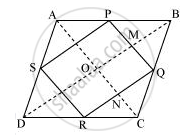# ABCD is a rhombus and P, Q, R and S are the mid-points of the sides AB, BC, CD and DA respectively. Show that the quadrilateral PQRS is a rectangle. - Mathematics

ABCD is a rhombus and P, Q, R and S are the mid-points of the sides AB, BC, CD and DA respectively. Show that the quadrilateral PQRS is a rectangle.

#### SolutionIn ΔABC, P and Q are the mid-points of sides AB and BC respectively.

∴ PQ || AC and PQ = 1/2AC (Using mid-point theorem) ... (1)

R and S are the mid-points of CD and AD respectively.

∴ RS || AC and RS = 1/2AC (Using mid-point theorem) ... (2)

From equations (1) and (2), we obtain

PQ || RS and PQ = RS

Since in quadrilateral PQRS, one pair of opposite sides is equal and parallel to

each other, it is a parallelogram.

Let the diagonals of rhombus ABCD intersect each other at point O.

MQ || ON (∵ PQ || AC)

QN || OM (∵ QR || BD)

Therefore, OMQN is a parallelogram.

⇒ ∠MQN = ∠NOM

⇒ ∠PQR = ∠NOM

However, ∠NOM = 90° (Diagonals of a rhombus are perpendicular to each other)

∴ ∠PQR = 90°

Clearly, PQRS is a parallelogram having one of its interior angles as 90º.

Hence, PQRS is a rectangle.

Concept: The Mid-point Theorem
Is there an error in this question or solution?

#### APPEARS IN

NCERT Class 9 Maths